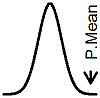P.Mean: Reviewing how the binomial and negative binomial distributions work (created 2012-05-17). News: Sign up for "The Monthly Mean," the newsletter that dares to call itself average, www.pmean.com/news.When you look at the binomial distribution and the negative binomial distribution side by side, they look almost identical. But the subtle differences are important. I was working on some problems involving these two distributions and thought it might be helpful to review their properties. These properties are indeed well known, but I wanted to get comfortable with them before I started tackling some more complex alternatives to these two distributions.

The binomial distribution is a model for the number of successes in a fixed number of trials. The number of successes is bounded below by zero and above by the number of trials. Here is the formula for the probability of observing j successes.whereThis is often read as "n choose j" or "combination of n things taken j at a time." Now let's contrast the binomial probability to the probability of a negative binomial.

The negative binomial distribution is model for the number of failures that you have to endure before you get a specific number of successes. That's one subtle difference right away. The number of successes is random for the binomial case and fixed for the negative binomial case. The other subtle difference is that while both the binomial and negative binomial distributions are bounded below by zero, the negative binomial has no firm upper bound. The probability that a number of failures observed is j has the following formula.We've got the same powers of pi and 1-pi, or so it seems. But the difference here is that the number of successes in a negative binomial distribution does not go down as the number of failures go up. Also another subtle difference is that the combination formula used in the negative binomial is one smaller on the top and one smaller on the bottom. These differences are actually much more apparent when you list all of the probabilities. Consider a simple case with n=2 for the binomial and r=2 for the negative binomial. In the first case, the probabilities areand in the second case the probabilities areIt may help to visualize these coefficients using Pascal's triangle.The entries in Pascal's triangle are the various combinations. Notice that any entry in Pascal's triangle is the sum of the two terms above and to the left and above and to the right. So the 15 in the bottom row of this triangle is the sum of the 5 above and to the left and the 10 above and to the right. This establishes the well known factor thatThe coefficients for a binomial random variable correspond to a particular row of Pascal's triangleand the coefficients for a negative binomial random variable correspond to a diagonal in Pascal's triangle.Wow that quite a shock. Look at how that combination increases so rapidly in the negative binomial case. And we have to sum up an infinite number of them as well. How could this ever sum up to only 1.0? Well, in both cases the probabilities do indeed sum to 1.0 and it is not difficult to show how this works.

There's a trick that helps here. First demonstrate that the probabilities add up to 1 in the simplest case. Then make an assumption that the probabilities add up to 1 for an arbitrary value n. If that assumption allows you to demonstrate a similar finding for n+1, then you have proved your case for every value of n. This is called proof by induction.

Let's examine the simplest binomial case, with n=1. In that setting,Now, assume that the formula holds for n.Now multiply this sum by piReplace j with j-1.Take the original sum and multiply it by 1-pi instead.Add these two equations together. The second sum starts at 0 and the first sum ends at n+1, so these can't be combined. But everything between j=1 and j=n can be combined.The sum of the two combinations simplifies nicely, as shown earlier. It is also convenient to note thatSubstituting these into the binomial sum and using the relationship involving the adjacent combination, you getwhich simplifies toThere is a similar way that you can show that the sum of negative binomial probabilities equals 1. Start with the case r=1. This is a special case of the negative binomial distribution known as the geometric distribution.This is a geometric progression. There is a standard formula that you can apply here, but I won't show the details. Now, assume that the negative binomial sums to 1 for the case r=n.Multiply the left side by that geometric progression, which is just the same as multiplying by one.Re-arrange terms to getNow, you can't just cavalierly rearrange terms in an infinite sum. So you have to do a bit of justification here, which I won't show (at least for now). That inside sum simplifies much as above. Consider the first two terms.based on the previously established relationship. Now add in the third termThe same pattern holds all the way down. When you are finished, you getwhich is the negative binomial distribution with n+1 instead of n.

It may help to view these relationships using Pascal's triangle. Here's Pascal's triangle with the first four combination coefficients for a particular negative binomial highlighted.What is the sum of these four coefficients? First, let's swap one of the values.That doesn't change the sum, does it? Now notice that the sum of the 1 and the 3 appears beneath and between those two numbers.Now the sum of the 6 and the 4 appears beneath and between those two numbers.Finally, the sum of the two 10's appears beneath and between.It's pretty cool how those sums cascade down Pascal's triangle.This page was written by Steve Simon and is licensed under the Creative Commons Attribution 3.0 United States License. Need more information? I have a page with general help resources. You can also browse for pages similar to this one at Statistical Theory.